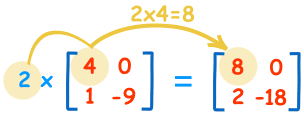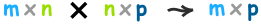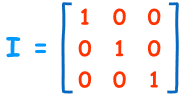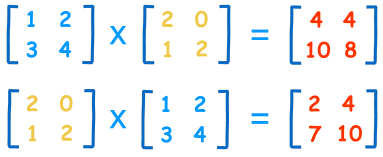# How to Multiply Matrices

A Matrix is an array of numbers:A Matrix
(This one has 2 Rows and 3 Columns)

To multiply a matrix by a single number is easy:These are the calculations:
 2×4=8 2×0=0 2×1=2 2×-9=-18

We call the number ("2" in this case) a scalar, so this is called "scalar multiplication".

## Multiplying a Matrix by Another Matrix

But to multiply a matrix by another matrix we need to do the "dot product" of rows and columns ... what does that mean? Let us see with an example:

To work out the answer for the 1st row and 1st column:The "Dot Product" is where we multiply matching members, then sum up:

(1, 2, 3) • (7, 9, 11) = 1×7 + 2×9 + 3×11
= 58

We match the 1st members (1 and 7), multiply them, likewise for the 2nd members (2 and 9) and the 3rd members (3 and 11), and finally sum them up.

Want to see another example? Here it is for the 1st row and 2nd column:(1, 2, 3) • (8, 10, 12) = 1×8 + 2×10 + 3×12
= 64

We can do the same thing for the 2nd row and 1st column:

(4, 5, 6) • (7, 9, 11) = 4×7 + 5×9 + 6×11
= 139

And for the 2nd row and 2nd column:

(4, 5, 6) • (8, 10, 12) = 4×8 + 5×10 + 6×12
= 154

And we get:DONE!

## Why Do It This Way?

This may seem an odd and complicated way of multiplying, but it is necessary!

I can give you a real-life example to illustrate why we multiply matrices in this way.

### Example: The local shop sells 3 types of pies.

• Apple pies cost \$3 each
• Cherry pies cost \$4 each
• Blueberry pies cost \$2 each

And this is how many they sold in 4 days:Now think about this ... the value of sales for Monday is calculated this way:

Apple pie value + Cherry pie value + Blueberry pie value
\$3×13 + \$4×8 + \$2×6 = \$83

So it is, in fact, the "dot product" of prices and how many were sold:

(\$3, \$4, \$2) • (13, 8, 6) = \$3×13 + \$4×8 + \$2×6
= \$83

We match the price to how many sold, multiply each, then sum the result.

In other words:

• The sales for Monday were: Apple pies: \$3×13=\$39, Cherry pies: \$4×8=\$32, and Blueberry pies: \$2×6=\$12. Together that is \$39 + \$32 + \$12 = \$83
• And for Tueday: \$3×9 + \$4×7 + \$2×4 = \$63
• And for Wednesday: \$3×7 + \$4×4 + \$2×0 = \$37
• And for Thursday: \$3×15 + \$4×6 + \$2×3 = \$75

So it is important to match each price to each quantity.

Now you know why we use the "dot product".

And here is the full result in Matrix form:They sold \$83 worth of pies on Monday, \$63 on Tuesday, etc.

(You can put those values into the Matrix Calculator to see if they work.)

## Rows and Columns

To show how many rows and columns a matrix has we often write rows×columns.

Example: This matrix is 2×3 (2 rows by 3 columns):When we do multiplication:

• The number of columns of the 1st matrix must equal the number of rows of the 2nd matrix.
• And the result will have the same number of rows as the 1st matrix, and the same number of columns as the 2nd matrix.

### Example:In that example we multiplied a 1×3 matrix by a 3×4 matrix (note the 3s are the same), and the result was a 1×4 matrix.

In General:

To multiply an m×n matrix by an n×p matrix, the ns must be the same,
and the result is an m×p matrix.## Identity Matrix

The "Identity Matrix" is the matrix equivalent of the number "1":A 3×3 Identity Matrix

• It is "square" (has same number of rows as columns)
• It can be large or small (2×2, 100×100, ... whatever)
• It has 1s on the diagonal and 0s everywhere else
• Its symbol is the capital letter I

It is a special matrix, because when we multiply by it, the original is unchanged:

A × I = A

I × A = A

## Order of Multiplication

In arithmetic we are used to:

3 × 5 = 5 × 3
(The Commutative Law of Multiplication)

But this is not generally true for matrices (matrix multiplication is not commutative):

AB ≠ BA

When we change the order of multiplication, the answer is (usually) different.

### Example:

See how changing the order affects this multiplication:The answers are different!

It can have the same result (such as when one matrix is the Identity Matrix) but not usually.